Tutorials
+4

Experts' Favorite Data Science Techniques

What are the most favorite techniques of the professional data scientists interviewed in DataFramed, a DataCamp podcast? Explore all 6 of them in this tutorial!

We have recently launched a new data science podcast called DataFramed, in which I speak with experts and thought leaders from academia and industry about what data science looks like in practice and how it's changing society. I often ask my guests what one of their data science techniques is.

This tutorial, which is a write-up of a Facebook Live event we did a week ago, will take you through a bunch of them!

In this tutorial, you'll look at

You can subscribe to DataFramed on iTunes here and on the Google play store here.

Give-away

We're also having a give-away for those who write iTunes reviews for us! 5 lucky randomly selected reviewers will receive DataCamp swag: we've got sweatshirts, pens, stickers, you name it and one of those 5 will be selected to interview me in one of our podcast segments!

What do you need to do?

• Write a review of DataFramed in the iTunes store
• email [email protected] a screenshot of the review and the country in whose store you posted it (note: this email address is not regularly checked except for this give-away).
• Do these things by EOD Friday March 9th in your time zone.

Scatter plots

Roger Peng appeared on last week's episode of DataFramed. Roger is a Professor in the Department of Biostatistics at the Johns Hopkins Bloomberg School of Public Health, co-director of the Johns Hopkins Data Science Lab and co-founder of the Johns Hopkins Data Science Specialization. Roger is also a well-seasoned podcaster on Not so Standard deviations and the Effort Report. In this episode, we talked about data science, it's role in researching the environment and air pollution, massive open online courses for democratizing data science and much more.

In Roger's words,

Frankly, my favorite tool is just simply a scatter plot. I think plotting is so revealing. It's not something that, frankly, I see a lot done. I think the reason why, I thought about why this is the case, and I think the reason is because it's one of those tools that really instills trust in the people who receive the plot. Because they feel like they can see the data, they feel like they can understand if you have a model that's overlaid they know how the data goes into the model. They can reason about the data and I think it's one of the really critical things for building trust.

So let's now build some scatter plots to see their power. First you'll import some required packages, import your data and check it out.

Note that packages such as numpy, pandas, matplotlib and seaborn usually are imported with an alias so that you don't always need to type the entire package name, just like in the code chunk below:

# Import packages
import numpy as np
import pandas as pd
import matplotlib.pyplot as plt
import seaborn as sns
% matplotlib inline
sns.set()

Remember that Matplotlib will generate figures but ideally, you don't want to send them to a file. You want them to appear inline in your notebook, so you some IPython magic to get this: % matplotlib inline.

Now, you're ready to import some data and inspect the first couple of rows of the DataFrame wit the .head() method:

# Import data and check out several rows
mean radius mean texture mean perimeter mean area mean smoothness mean compactness mean concavity mean concave points mean symmetry mean fractal dimension ... worst texture worst perimeter worst area worst smoothness worst compactness worst concavity worst concave points worst symmetry worst fractal dimension target
0 17.99 10.38 122.80 1001.0 0.11840 0.27760 0.3001 0.14710 0.2419 0.07871 ... 17.33 184.60 2019.0 0.1622 0.6656 0.7119 0.2654 0.4601 0.11890 0
1 20.57 17.77 132.90 1326.0 0.08474 0.07864 0.0869 0.07017 0.1812 0.05667 ... 23.41 158.80 1956.0 0.1238 0.1866 0.2416 0.1860 0.2750 0.08902 0
2 19.69 21.25 130.00 1203.0 0.10960 0.15990 0.1974 0.12790 0.2069 0.05999 ... 25.53 152.50 1709.0 0.1444 0.4245 0.4504 0.2430 0.3613 0.08758 0
3 11.42 20.38 77.58 386.1 0.14250 0.28390 0.2414 0.10520 0.2597 0.09744 ... 26.50 98.87 567.7 0.2098 0.8663 0.6869 0.2575 0.6638 0.17300 0
4 20.29 14.34 135.10 1297.0 0.10030 0.13280 0.1980 0.10430 0.1809 0.05883 ... 16.67 152.20 1575.0 0.1374 0.2050 0.4000 0.1625 0.2364 0.07678 0

5 rows × 31 columns

In this case, you have imported the Breast Cancer (Wisconsin) data set. But, before you jump into scatterplots, it's always good to check what the column names, types and how many entries you have in your DataFrame df with the .info() method:

df.info()
<class 'pandas.core.frame.DataFrame'>
RangeIndex: 569 entries, 0 to 568
Data columns (total 31 columns):
mean texture               569 non-null float64
mean perimeter             569 non-null float64
mean area                  569 non-null float64
mean smoothness            569 non-null float64
mean compactness           569 non-null float64
mean concavity             569 non-null float64
mean concave points        569 non-null float64
mean symmetry              569 non-null float64
mean fractal dimension     569 non-null float64
texture error              569 non-null float64
perimeter error            569 non-null float64
area error                 569 non-null float64
smoothness error           569 non-null float64
compactness error          569 non-null float64
concavity error            569 non-null float64
concave points error       569 non-null float64
symmetry error             569 non-null float64
fractal dimension error    569 non-null float64
worst texture              569 non-null float64
worst perimeter            569 non-null float64
worst area                 569 non-null float64
worst smoothness           569 non-null float64
worst compactness          569 non-null float64
worst concavity            569 non-null float64
worst concave points       569 non-null float64
worst symmetry             569 non-null float64
worst fractal dimension    569 non-null float64
target                     569 non-null int64
dtypes: float64(30), int64(1)
memory usage: 137.9 KB

You get all kinds of information from executing this line of code. You see, among other things, that you have a lot of numerical data. In that case, you might also want to use .describe() to check out summary statistics of the columns:

df.describe()
mean radius mean texture mean perimeter mean area mean smoothness mean compactness mean concavity mean concave points mean symmetry mean fractal dimension ... worst texture worst perimeter worst area worst smoothness worst compactness worst concavity worst concave points worst symmetry worst fractal dimension target
count 569.000000 569.000000 569.000000 569.000000 569.000000 569.000000 569.000000 569.000000 569.000000 569.000000 ... 569.000000 569.000000 569.000000 569.000000 569.000000 569.000000 569.000000 569.000000 569.000000 569.000000
mean 14.127292 19.289649 91.969033 654.889104 0.096360 0.104341 0.088799 0.048919 0.181162 0.062798 ... 25.677223 107.261213 880.583128 0.132369 0.254265 0.272188 0.114606 0.290076 0.083946 0.627417
std 3.524049 4.301036 24.298981 351.914129 0.014064 0.052813 0.079720 0.038803 0.027414 0.007060 ... 6.146258 33.602542 569.356993 0.022832 0.157336 0.208624 0.065732 0.061867 0.018061 0.483918
min 6.981000 9.710000 43.790000 143.500000 0.052630 0.019380 0.000000 0.000000 0.106000 0.049960 ... 12.020000 50.410000 185.200000 0.071170 0.027290 0.000000 0.000000 0.156500 0.055040 0.000000
25% 11.700000 16.170000 75.170000 420.300000 0.086370 0.064920 0.029560 0.020310 0.161900 0.057700 ... 21.080000 84.110000 515.300000 0.116600 0.147200 0.114500 0.064930 0.250400 0.071460 0.000000
50% 13.370000 18.840000 86.240000 551.100000 0.095870 0.092630 0.061540 0.033500 0.179200 0.061540 ... 25.410000 97.660000 686.500000 0.131300 0.211900 0.226700 0.099930 0.282200 0.080040 1.000000
75% 15.780000 21.800000 104.100000 782.700000 0.105300 0.130400 0.130700 0.074000 0.195700 0.066120 ... 29.720000 125.400000 1084.000000 0.146000 0.339100 0.382900 0.161400 0.317900 0.092080 1.000000
max 28.110000 39.280000 188.500000 2501.000000 0.163400 0.345400 0.426800 0.201200 0.304000 0.097440 ... 49.540000 251.200000 4254.000000 0.222600 1.058000 1.252000 0.291000 0.663800 0.207500 1.000000

8 rows × 31 columns

Besides asking yourself some questions about the data you're working with, you should also ask yourself "what is the data"?

All too often, people jump into data sets without knowing where the data comes from, how it was collected, what the data lineage is, etc. Check out the full description of the data set that you just imported here.

Why are you looking at this data set? You should also think about the end goal that you're trying to accomplish with your data analysis!

In this case, one potential answer to this is that you're looking at this data to build a model to predict a diagnosis. Can you, based on the data, say whether a tumor is benign or malignant?

Now it's time to build some plots! Let's build a scatter plot of the first two features in your data set, namely, mean radius and mean texture. The best thing about this is that you can use the Pandas plotting methods to do this natively!

For the x argument, you want to pass in the column name 'mean radius'. Similarly, you'll pass 'mean texture' as the y argument:

# Scatter plot of 1st two features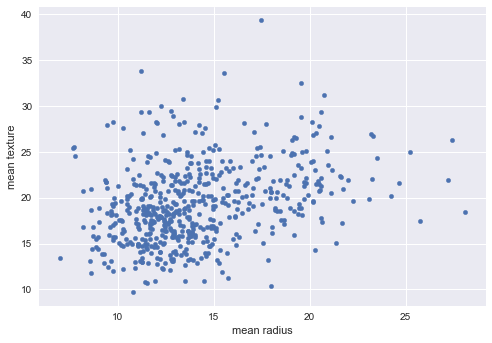Tip: suppress the text output from Matplotlib with the semicolon ;.

You can see that there is some sort of correlation: as the mean radius increases, also the mean texture goes up slightly. It's a bit sparse towards the right-hand side of the plot. At this point, you're not sure whether it's linear or not, but it's definitely not highly non-linear. That means that if you would want to do a linear model on the data, that could suffice. However, the point is that you can just look at it and think about what you're seeing and put that against the original question you asked yourself.

In this case, you're working with the question of prediction whether a tumor is benign or malignant. In such cases, you might want to have the scatter plot colored by the target. You can do this by adding the argument c and setting it to 'target'.

# Scatter plot colored by 'target'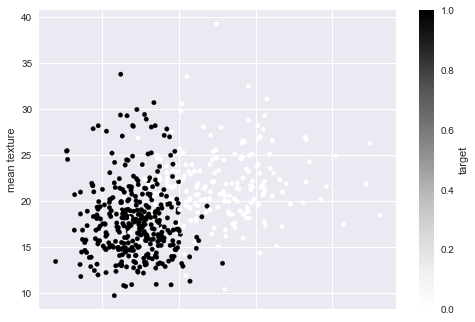You see that there are two clear clusters now in the plot. That's a sign that these two features of your data set are already pretty great at classifying tumors! This is a valuable insight already!

Let's discover some more scatter plots. Earlier in this tutorial, you discovered that you couldn't really be sure of the linearity of your data. This is the perfect opportunity for you to make a scatter plot with linear regression, just like you do in the following code chunk:

# Scatter plot with linear regression
<seaborn.axisgrid.FacetGrid at 0x108bec390>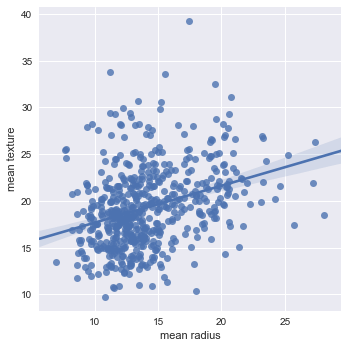In this case, you use Seaborn's lmplot() to plot the 'mean radius' and 'mean texture'. Note also that you have to pass in df as an argument to the plotting function. You now see that the linear regression goes through the plot. The small areas that you see appearing next to the line indicate the confidence bounds. From here on out, you could calculate the slope, see how the texture increases with radius, etc.

However, now, you're going to do the same, but you're going to color it by target, which is the diagnosis:

# Scatter plot colored by 'target' with linear regression
sns.lmplot(x='mean radius', y='mean texture', hue='target', data=df)
<seaborn.axisgrid.FacetGrid at 0x108ab34a8>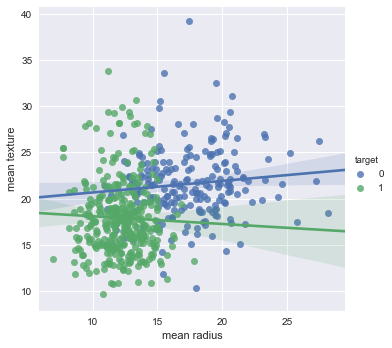You now see the two subsets corresponding to target 1 and target 0 and the different linear regressions. Now, for target 0, you have a positive linear regression, whereas for target 1, you have a linear regression with a negative slope. That might not be statistically significant but you see the confidence bounds are actually quite large on the right-hand side of the plot.

You've already learned a great deal about your data set, but let's now check out some other features that you think may be related, such as 'mean radius' and 'mean perimeter':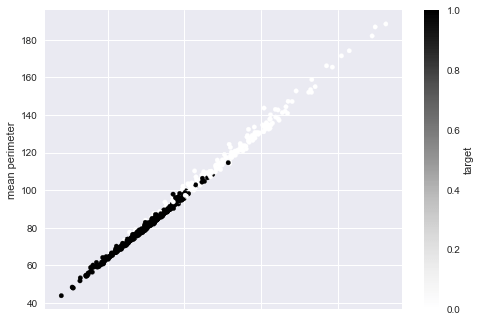You see that there's a rather sharp division between the benign and malignant tumors, and on top of that, you see that there's pretty much a straight line. In this data set, 'mean radius' and 'mean perimeter' are highly correlated.

What does that mean for you?

If you want to use a Machine Learning model afterwards, it means that you might do well just using one of both features, since the information in one is contained within the other.

But why is this important?

It might not be important for a data set like this, that isn't really all that big, but it might be for when you're working with big data with hundreds of thousands of features. In such cases, you might want to reduce the number of dimensions of the data. This is called "dimensionality reduction".

Also check out 'mean radius' versus 'mean area':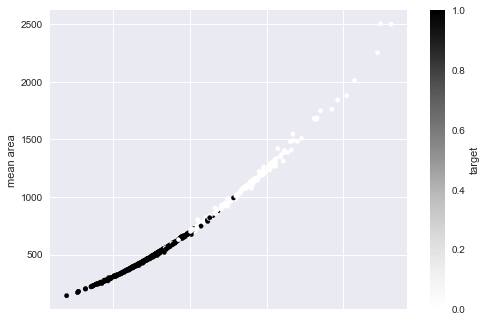When you look at this plot, you wouldn't want to model this using a linear regression, for example. Once again, you see that scatterplots give a huge deal of information about the modeling techniques that you might (not) want to use.

You're now going to build a pairplot of this dataset. That means that this plot will contain all possible scatter plots of all features, with histograms along the diagonal. But first you'll subset the data to return the first four and the final column:

df_sub = df.iloc[:,[0,1,2,3,-1]]

Now it is time to build you pairplot, using seaborn!

sns.pairplot(df_sub, hue='target', size=5);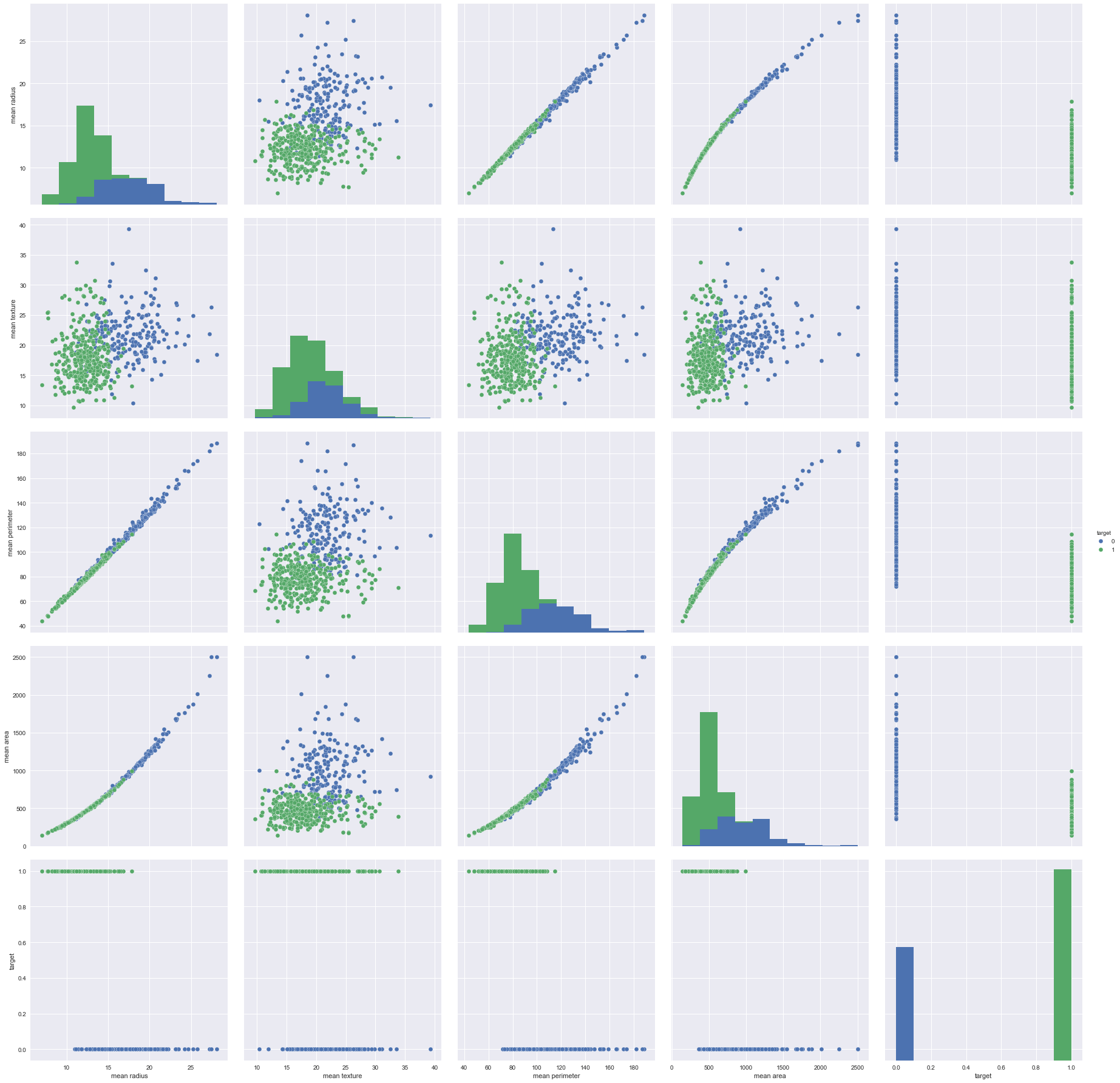Do you now understand why Roger Peng loves scatter plots? With these plots, you an extract a ton of information from your data set already and gather some interesting insights as to what modeling techniques you might (not) want to be using.

Up next: you'll discover the machine learning super power of decision trees. But whose favorite data science technique is this?

Decision trees for prediction

In episode 2 of DataFramed, I spoke with Chris Volinksy, Assistant Vice President for Big Data Research at AT&T Labs, and all around top bloke. What he said was:

I'm always amazed at the power of some of the old school techniques. Good old fashioned linear regression is still a really powerful and interpretable, and tried and true technique. It's not always appropriate, but often works well. Decision trees are another old school technique, I'm always amazed at how well they work. But, you know, one thing I always find really powerful are well done, well-thought out data visualizations. And, you know, I'm a big fan of the type of data visualization that I see in media companies.

You've already done some data visualizations, you'll soon do some linear regression. Now, you're going to build a decision tree. And you're going to build a decision tree classifier.

So: what is a decision tree classifier?

It is a tree that allows you to classify data points (also known as "target variables", for example, benign or malignant tumor) based on feature variables (such as geometric measurements of tumors). Take a look at this example: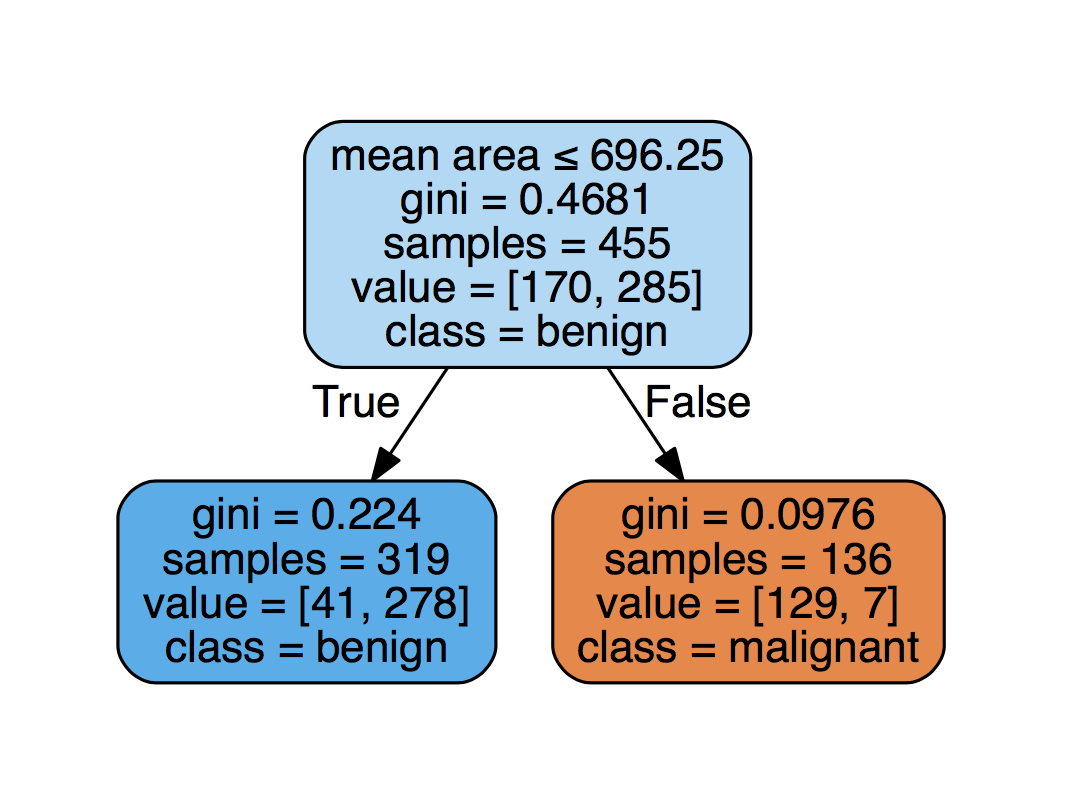But why do DataFramed's podcast guests like these? Because they are interpretable! Another way of saying this is

An interpretable model is one whose predictions you can explain.

This is a direct verbatim quotation by Mike Lee Williams, Research Engineer, Cloudera Fast Forward Labs (check out this segment where he talks about machine learning interpretability).

You first fit such a model to your training data, which means deciding (based on the training data) which decisions will split at each branching point in the tree. For example, fitting it will decide that the first branch is on the feature 'mean area' and that 'mean area' less than 696.25 results in a prediction of 'benign'.

Note that it's actually the Gini coefficient which is used to make these decisions. At this point, you won't delve deeper into this.

So let's now build a decision tree classifier. First up, you'll create numpy arrays X and y that contain your features and your target, respectively:

X = df_sub.drop('target', axis=1).values
y = df_sub['target'].values

Next, you'll want to go through the following steps:

• You'll want to fit (or train) your model on a subset of the data, called the training set.
• You'll then test it on the another set, the test set. Testing means that you'll predict on that set and see how good the predictions are.
• You'll use a metric called accuracy, which is the fraction of correct predictions.

However, first, you need to split your data in training/test sets using scikit-learn:

from sklearn.model_selection import train_test_split
X_train, X_test, y_train, y_test = train_test_split(X, y, test_size=0.2,
random_state=42, stratify=y)

Note that you specify the stratify argument so that you keep the proportion of different values of y in the test as well as the training data.

Now, you get to build your decision tree classifier. First create such a model with max_depth=2 and then fit it your data:

from sklearn import tree
clf = tree.DecisionTreeClassifier(max_depth=2)
clf.fit(X_train, y_train)
DecisionTreeClassifier(class_weight=None, criterion='gini', max_depth=2,
max_features=None, max_leaf_nodes=None,
min_impurity_split=1e-07, min_samples_leaf=1,
min_samples_split=2, min_weight_fraction_leaf=0.0,
presort=False, random_state=None, splitter='best')

Next, you can compute the accuracy on the test set with the .score() method:

clf.score(X_test, y_test)
0.89473684210526316

For fun, you can also compute the score on the training set:

clf.score(X_train, y_train)
0.90109890109890112

The classifier performs better on the training data but that's because you used this set to build your classifier in the first place.

Lastly, visualize your decision tree using graphviz:

import graphviz
dot_data = tree.export_graphviz(clf, out_file=None,
feature_names=df_sub.drop('target', axis=1).columns,
class_names=['malignant', 'benign'],
filled=True, rounded=True,
special_characters=True)
graph = graphviz.Source(dot_data)
graph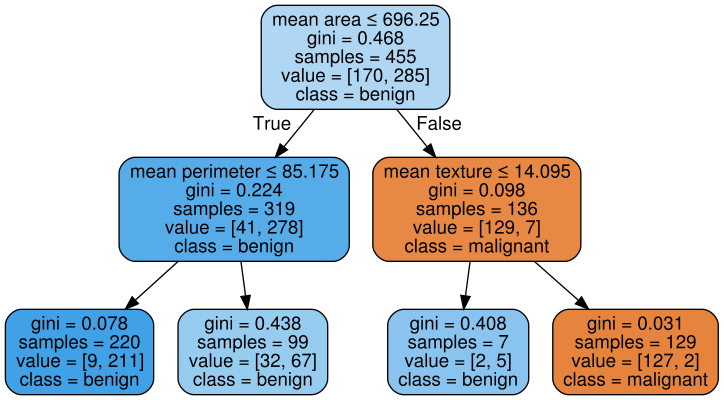Linear regression

You already saw that Chris Volinsky was a huge fan of linear regression:

But, I'm always amazed at the power of some of the old school techniques. Good old fashioned linear regression is still a really powerful and interpretable, and tried and true technique.

The above tumor prediction task was a classification task, you were trying to classify tumors.

The other well-known prediction task is called a regression task, in which you're trying to predict a numeric quantity, such as the life expectancy in a given nation.

Let's import some Gapminder data to do so:

# Import data and check out first rows
population fertility HIV CO2 BMI_male GDP BMI_female life child_mortality Region
0 34811059.0 2.73 0.1 3.328945 24.59620 12314.0 129.9049 75.3 29.5 Middle East & North Africa
1 19842251.0 6.43 2.0 1.474353 22.25083 7103.0 130.1247 58.3 192.0 Sub-Saharan Africa
2 40381860.0 2.24 0.5 4.785170 27.50170 14646.0 118.8915 75.5 15.4 America
3 2975029.0 1.40 0.1 1.804106 25.35542 7383.0 132.8108 72.5 20.0 Europe & Central Asia
4 21370348.0 1.96 0.1 18.016313 27.56373 41312.0 117.3755 81.5 5.2 East Asia & Pacific

Check out the column names, types and how many entries there are in your DataFrame df_gm:

df_gm.info()
<class 'pandas.core.frame.DataFrame'>
RangeIndex: 139 entries, 0 to 138
Data columns (total 10 columns):
population         139 non-null float64
fertility          139 non-null float64
HIV                139 non-null float64
CO2                139 non-null float64
BMI_male           139 non-null float64
GDP                139 non-null float64
BMI_female         139 non-null float64
life               139 non-null float64
child_mortality    139 non-null float64
Region             139 non-null object
dtypes: float64(9), object(1)
memory usage: 10.9+ KB

You're going to use a linear regression model to try to predict the life expectancy in a given country, based on its fertility rate. But first, make a scatter plot ;)

df_gm.plot.scatter(x='fertility', y='life');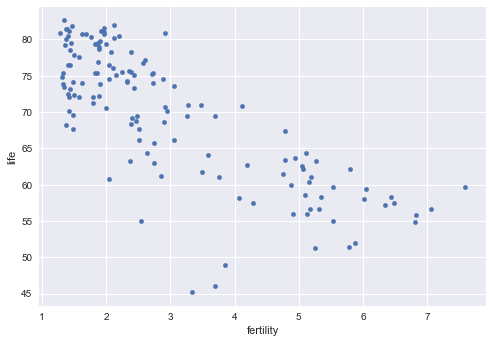But exactly can you expect from a linear model? A linear model fits a straight line to the data:

$y = a_0 + a_1x.$

This is once again an interpretable model as it tells you that a 1-unit increase in $x$ leads to an $a_1$ increase in $y$.

Let's now see this in action. You'll fit the model to the entire data set and visualize the regression. Note that fitting the model determines the parameters $a_i$ in the above equation:

# Subset data into feature and target
X_fertility = df_gm[['fertility']].values
y = df_gm[['life']].values
# Import LinearRegression
from sklearn.linear_model import LinearRegression

# Create the regressor: reg
reg = LinearRegression()

# Fit the model to the data
reg.fit(X_fertility, y)

# Plot scatter plot of data
plt.scatter(X_fertility, y)

# Create the prediction space
prediction_space = np.linspace(min(X_fertility), max(X_fertility)).reshape(-1,1)

# Compute predictions over the prediction space: y_pred
y_pred = reg.predict(prediction_space)

# Plot regression line
plt.plot(prediction_space, y_pred, color='black', linewidth=3);

# Print R^2
print(reg.score(X_fertility, y))
0.619244216774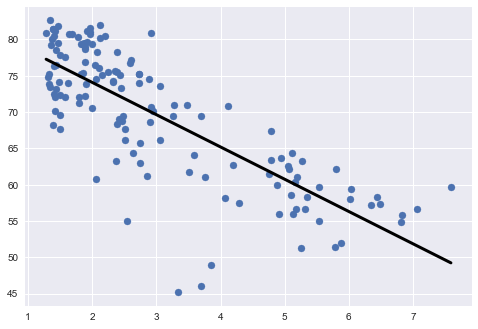Even before you think about interpreting this model by using equations to get out the coefficients, let's take a look at the line that is plotted. If you have a 1 unit increasing fertility, you have a 4.5 decrease in life expectancy. This is just an estimate, though.

To get it absolutely precise, you should print out the regression coefficient from the model:

# Print regression coefficient(s)
print(reg.coef_)
[[-4.44387899]]

Note: you'll generally want to normalize your data before using regression models and you may want to used a penalized regression such as lasso or ridge regression. See DataCamp's Supervised Learning with scikit-learn course for more on these techniques.

Now you can do the same using a two-parameter model, using 'fertility' and 'GDP':

$y = a_0 + a_1x_1 + a_2x_2.$

# Extract features from df_gm:
X = df_gm[['fertility', 'GDP']].values
# Create the regressor: reg
reg = LinearRegression()

# Fit the model to the data
reg.fit(X, y)

# Print R^2
print(reg.score(X, y))
0.692183492346
# Print regression coefficient(s)
print(reg.coef_)
[[ -3.55717843e+00   1.48369027e-04]]

Interpret the above regression coefficients.

But hold up. You didn't plot 'GDP'. What does it look like against 'life'. Plot it now to find out:

df_gm.plot.scatter(x='GDP', y='life');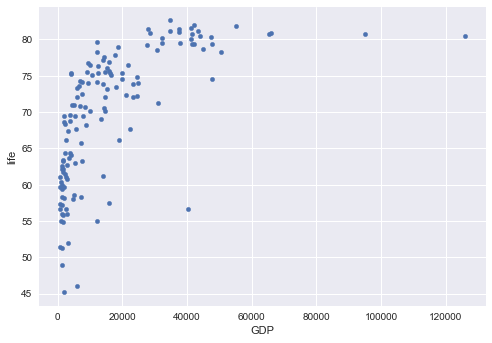This is definitely not linear! But this plot is still very interesting: the GDP is pretty bunched up between 0 and 40K but there are also values > 100,000. Are there plotting techniques to deal with this? The answer to this question is actually plotting the data with log axes. You'll find out more about this data science technique in the next section!

Plotting with Log Axes

In episode 6 of DataFramed, I interviewed David Robinson, Chief Data Scientist at DataCamp, about Citizen Data Science. Dave's favorite technique is using log axes.

So this is a simple technique, but it's one that I think is really underrated and is really kind of one of my favorites. It's learn to put something on a log scale. That is, take it from numbers that go one, two, three, four, five, six and if you can just instead have a scale that goes 1, 10, 100, 1,000. So that's really important when grafting because so many sets of numbers that we work with in the real world exist on scales that are much larger. That are these multiple different orders of magnitude.

Now, plot 'life' versus 'GDP' again, but this time with a log axis for 'GDP':

df_gm.plot.scatter(x='GDP', y='life');
plt.xscale('log')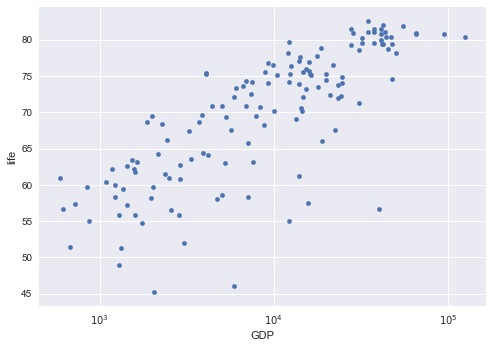That looks a whole lot better, doesn't it? Now, you get a far more valuable figure of your data that allows you to interpret it more correctly.

Logistic Regression

In episode 3 of DataFramed, I interviewed Claudia Perlich, Chief Scientist at Dstillery, where she led the machine learning efforts that help target consumers and derive insights for marketers. We spoke about the role of data science in the online advertising world, the predictability of humans, how Claudia's team built real-time bidding algorithms and detected bots online, along with the ethical implications of all of these evolving concepts.

Today I really value the simplicity and elegance and also transparency that you can get from linear models like logistic regression ... because it's so much easier to look under the hood and understand what might be going on there. It really has become my go to tool over the last I would say 10, 15 years. In fact, I won all of my data mining competitions using some form of a logistic model.

Now, before you get started, let's clarify something: logistic regression is a linear classification algorithm. In this section, you'll use a logistic regression model to build classification predictions for the breast cancer dataset.

How Does Logistic Regression Work?

Logistic regression essentially takes a linear combination of the features

$t = a_0 + a_1x_1 + a_2x_2 + \ldots + a_nx_n.$

Then transforms $t$ into

$p = \frac{1}{1+e^{-t}}.$

Let's now visualize this transformation $t \to p$:

t = np.linspace(-8,8,100)
p = 1/(1+np.exp(-t))
plt.plot(t,p);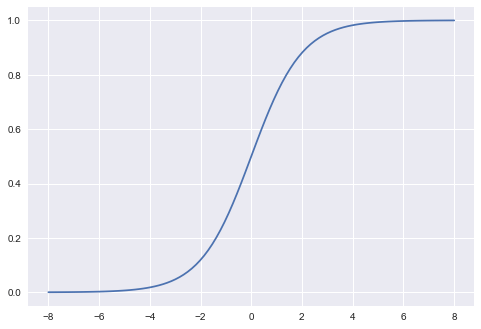You get this s-shaped function or a sigmoid function. So, if this linear combination is large, then $p$ gets closer to 1. On the other hand, if it's negative, $p$ gets closer to 0.

$p$ is the estimated probability that, for example, the tumor is malignant. In other words, if the sum gets really close to 1, the probability of the tumor being malignant is high. Similarly, a sum that is rather closer to 0 makes is more likely that the tumor is benign.

Note: fitting the model to the data determines the coefficients $a_i$.

If $p>0.5$, you classify the target as 1 (malignant), otherwise as 0 (benign).

How is This Model Interpretable?

Well, rearranging the above equations yield

$a_0 + a_1x_1 + a_2x_2 + \ldots + a_nx_n = t = \text{log}(\frac{p}{1-p})=\text{logit}(p)$

And $\frac{p}{1-p}$ is called the odds ratio: this is the probability of the tumor being malignant over the probability of the tumor being benign.

So: increasing $x_1$ by 1 unit will increase the odds ratio $\frac{p}{1-p}$ by $\text{exp}(a_1)$ units. It is in this way that logistic regression is interpretable. It tells you how the relative likelihood of benign versus malignant changes when these features change.

Let's see this in action.

# Check out 1st several rows of data for reacquaintance purposes
mean radius mean texture mean perimeter mean area target
0 17.99 10.38 122.80 1001.0 0
1 20.57 17.77 132.90 1326.0 0
2 19.69 21.25 130.00 1203.0 0
3 11.42 20.38 77.58 386.1 0
4 20.29 14.34 135.10 1297.0 0
# Split into features/target
X = df_sub.drop('target', axis=1).values
y = df_sub['target'].values
#Build logistic regression model, fit to training set
from sklearn import linear_model
logistic = linear_model.LogisticRegression()
logistic.fit(X_train, y_train)
LogisticRegression(C=1.0, class_weight=None, dual=False, fit_intercept=True,
intercept_scaling=1, max_iter=100, multi_class='ovr', n_jobs=1,
penalty='l2', random_state=None, solver='liblinear', tol=0.0001,
verbose=0, warm_start=False)
# Compute accuracy on test set
logistic.score(X_test, y_test)
0.91228070175438591

Now print coefficients of logistic regression model:

logistic.coef_
array([[ 4.52966038, -0.16215141, -0.49868125, -0.02446923]])

With these coefficients, you see that a 1-unit increase in 'mean texture' will result in logit(p) decreasing by 0.16. That means that the odds ratio decreases by exp(0.16) = 1.17 or changes by a factor of 0.85.

Now calculate this with the help of numpy:

print(np.exp(0.16))
print(1/np.exp(0.16))
1.17351087099
0.852143788966

Principal Component Analysis (PCA)

In episode 8 of DataFramed, I chatted with Jake VanderPlas, a data science fellow at the University of Washington's eScience Institute, where his work focuses on data-intensive physical science research in an interdisciplinary setting. In the Python world, Jake is the author of the Python Data Science Handbook, and is active in maintaining and/or contributing to several well-known Python scientific computing packages, including Scikit-learn, Scipy, Matplotlib, Astropy, Altair, and others.

My all-time favorite in machine learning is principal component analysis. I just think it’s like a Swiss army knife, you can do anything with it...When I was a grad student I quickly found that whenever I was going to my meeting with my thesis advisor and I had new data set or something to look at, the first question that he was going to ask me was, "Well, did you do PCA? "

PCA is an example of dimensionality reduction and a favorite way to do it for many working data scientists. It's important as many datasets have way too many features to put into a scalable machine learning pipeline, for example, and it helps you to reduce the dimensionality of your data while retaining as much information as possible.

Note that, in essence, PCA a form of compression.

Once again, plot 'mean radius' against 'mean perimeter':Now, why would you want to compress this data, that is, reduce to a lower-dimensional space?

Well, if you have lots of features and data, it can take a while to process all of it. That's why you might want to reduce the dimension of your data beforehand. This is also known as compression. As you have read above, PCA is a way to achieve this compression.

The idea is hte following: if features are correlated as they are above, you may have enough information if you throw one of them away.

There are two basic steps:

1. The first step of PCA is to decorrelate your data and this corresponds to a linear transformation of the vector space your data lie in;
2. The second step is the actual dimension reduction; what is really happening is that your decorrelation step (the first step above) transforms the features into new and uncorrelated features; this second step then chooses the features that contain most of the information about the data (you'll formalize this soon enough).

Visualize the PCA transformation that preserves number of features:

# Split original breast cancer data into features/target
X = df.drop('target', axis=1).values
y = df['target'].values
# Scale features
from sklearn.preprocessing import StandardScaler
ss = StandardScaler()
ar_tot = ss.fit_transform(df)

# Apply PCA
from sklearn.decomposition import PCA
model_tot = PCA()
transformed = model_tot.fit_transform(ar_tot)
print(transformed.shape)
plt.scatter(transformed[:,0], transformed[:,1], c=y);
(569, 31)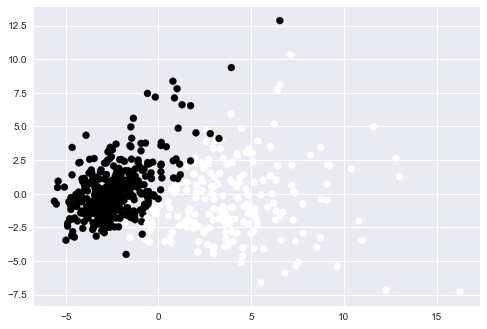Now plot the explained total variance of principal components against the number of components with the attribute explained_variance_ratio_:

plt.plot(np.cumsum(model_tot.explained_variance_ratio_));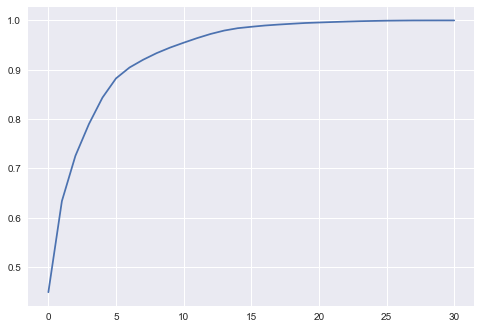The first five components explain nearly 90% of the total variation. In addition, in this plot, it looks like the first component explains as much as 40% of the variation. How much variance is contained in the first principal component?

model_tot.explained_variance_ratio_
0.44896035313223859

Now you're going to have some real fun by doing PCA before a logistic regression and seeing how many components you need to use to get the best model performance:

# Split original breast cancer data into features/target
X = df.drop('target', axis=1).values
y = df['target'].values
# Split data into test/train set
from sklearn.model_selection import train_test_split
X_train, X_test, y_train, y_test = train_test_split(X, y, test_size = 0.2, random_state=42, stratify=y)
# Build a pipeline of PCA w/ 20 components and a logistic regression
# NOTE: You should also scale your data; this will be an exercise for those
# eager ones out there
from sklearn.pipeline import Pipeline
pca = PCA(n_components=20)
pipe = Pipeline(steps=[('pca',pca), ('logistic', logistic)])
pipe.fit(X_train, y_train)
pipe.score(X_test, y_test)
0.96491228070175439

Now you're going to build a PCA/logistic regression pipeline for 1 component, 2 components and so on up to 30 components. You'll then plot accuracy as a function of the number of components used:

x1 = np.arange(1,30)
y1 = []
for i in x1:
pca = PCA(n_components=i)
pipe = Pipeline(steps=[('pca', pca), ('logistic', logistic)])
pipe.fit(X_train, y_train)
y1.append(pipe.score(X_test, y_test))
plt.plot(x1, y1);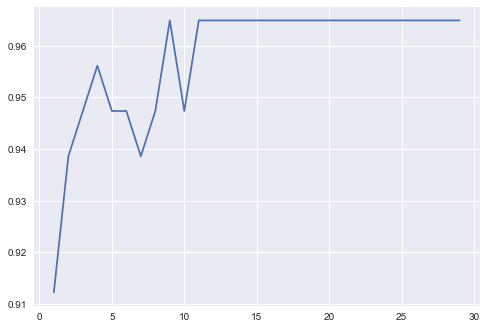The performance of this model increases as you increase the number of components. That is because you capture more of the variance as you add more components. Once you get 15 components, you get no better performance. That's why that number of components is enough to capture as much of the target as it can.

Conclusion

In this tutorial, you've imported a couple of datasets and explored them. Also, you have seen six favorite data science techniques explained:

• You have seen the power of scatter plots and why Roger Peng loves them so much.
• You've used the machine learning superpower of decision trees.
• You've used linear regression and and explored its interpretability.
• You've explored how log axes can make your plots easier to read.
• You've seen the power of logistic regression.
• You've checked out PCA, the swiss army knife of machine learning.

If you enjoyed this tutorial and the Facebook Live session, retweet or share on FB now and follow us on Twitter: @hugobowne and @DataCamp.